# RLine XLine AC Voltage Source Load Z Line = 2.4Ω + j 15Ω Fill in the...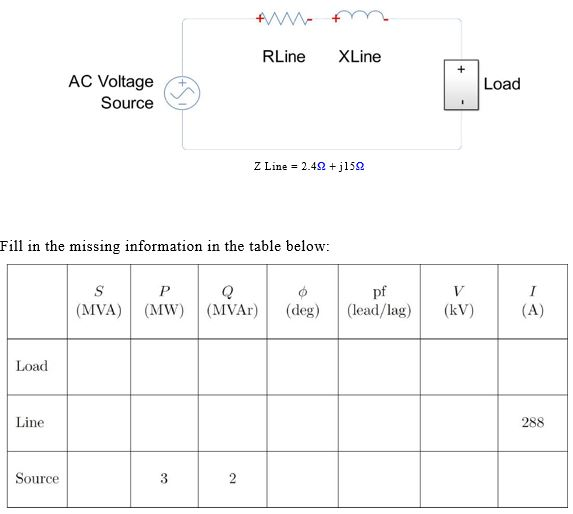RLine XLine AC Voltage Source Load Z Line = 2.4Ω + j 15Ω Fill in the missing information in the table below pf | (lead/lag)| (NIVA) | (MW) | (MVAr)| (deg) (kV) | (A) Load Line 288 ource 3

Given

Apparent Power delivered to the load S = 3 MW +j2 MVAR = 3.606 ∠33.69 MVA

Line current is 288, lets assume it as reference phasor Iline = 288 ∠0 Amp. This will be same for source and line since all are connected in series

We know

S = Vsource Iline*

Then load voltage is Vsource = S / Iline* = 3.606 ∠33.69 * 106/288 ∠0 = 12.519 kV ∠ 33.69

The source voltage leads the current by 33.69 degrees hence the power factor is cos(33.69) = 0.832 lagging

And load voltage is Vload = Vsource - Iline Zline = 12.519 kV ∠33.69 - 288∠0 x (2.4+j15) /1000 kV = 10.07 ∠15.1 kV

The load voltage leads the current by 15.1 degrees hence the power factor is cos(15.1) = 0.965 lagging

The load power can be thus calculated by

==> Sload = 10.07 ∠15.1 kV x 288∠0 = 2.9 ∠15.1 MVA = 2.8 MW + j 0.755 MVAR

Line power calculations:

Vline = I (R+jX) = 288 (2.4+j15) = 4.375 ∠80.9 kV

Pline = I2 R = 2882 x 2.4 = 199.065 kW

Qline = I2 X = 2882 x 15 = 1244.160 kVAR

Sline = 1260.27 ∠80.9 kVA = 1.26∠80.9 MVA

pf = cos 80.9 lag = 0.158 lag

Hence the completed table is :

 S (MVA) P(MW) Q(MVAR) Φ(deg) pf(lead/lag) V(kV) I(A) Load 2.9 2.8 0.755 15.1 0.965 lag 10.07 288 Line 1.26 0.199 1.244 80.9 0.158 lag 4.375 288 Source 3.606 3 2 33.69 0.832 lag 12.519 288
##### Add Answer to: RLine XLine AC Voltage Source Load Z Line = 2.4Ω + j 15Ω Fill in the...
Similar Homework Help Questions
• ### Consider a single-phase circuit where a 14 kV, 60 Hz voltage source feeds an electric load...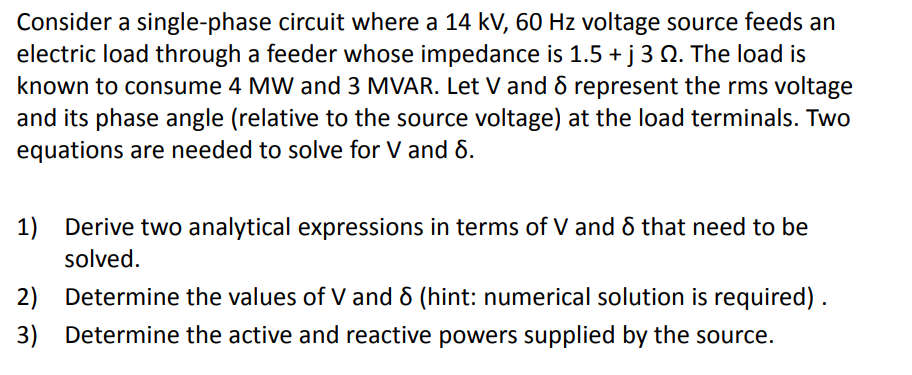Consider a single-phase circuit where a 14 kV, 60 Hz voltage source feeds an electric load through a feeder whose impedance is 1.5 + j 3 Ω. The load is known to consume 4 MW and 3 MVAR. Let V and δ represent the rms voltage and its phase angle (relative to the source voltage) at the load terminals. Two equations are needed to solve for V and δ. 1) Derive two analytical expressions in terms of V and δ...

• ### source + X mission line Resistance Resistive e Inductive Lead RS som i VST + R...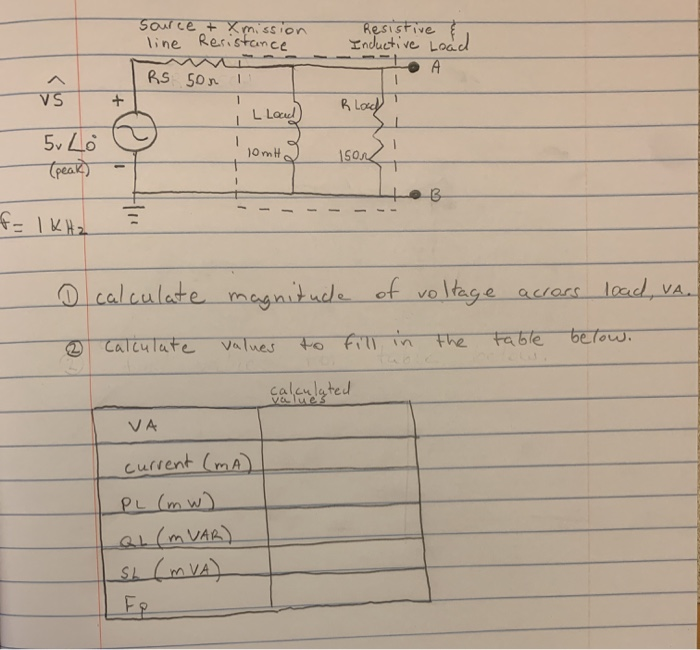source + X mission line Resistance Resistive e Inductive Lead RS som i VST + R loch " L Load 5v Lo Speak) o - FIKH = ------- o calculate magnitude of voltage across load, va. o calculate values to fill in the table below. calculated I VA current (mA) PL (mW). QL (MVAR) sb (m VA) FR

• ### 3. For the system shown below, determine the following (a) the reflection coefficient at the load end (b) the reflection coefficient at the source end (c) the VSWR (d) the phasor voltage at the load...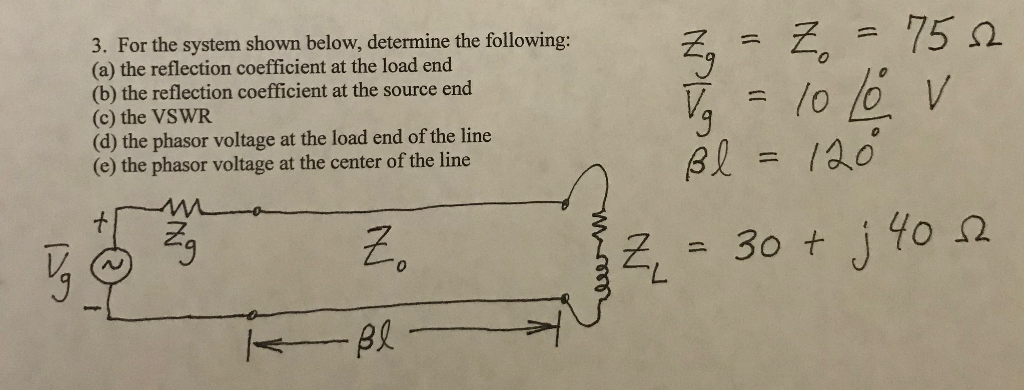3. For the system shown below, determine the following (a) the reflection coefficient at the load end (b) the reflection coefficient at the source end (c) the VSWR (d) the phasor voltage at the load end of the line (e) the phasor voltage at the center of the line 7-7=15Ω 0 3. For the system shown below, determine the following (a) the reflection coefficient at the load end (b) the reflection coefficient at the source end (c) the VSWR (d)...

• ### Review A circuit has an ac voltage source and a resistor and capacitor connected in series. There is no inductor. The a...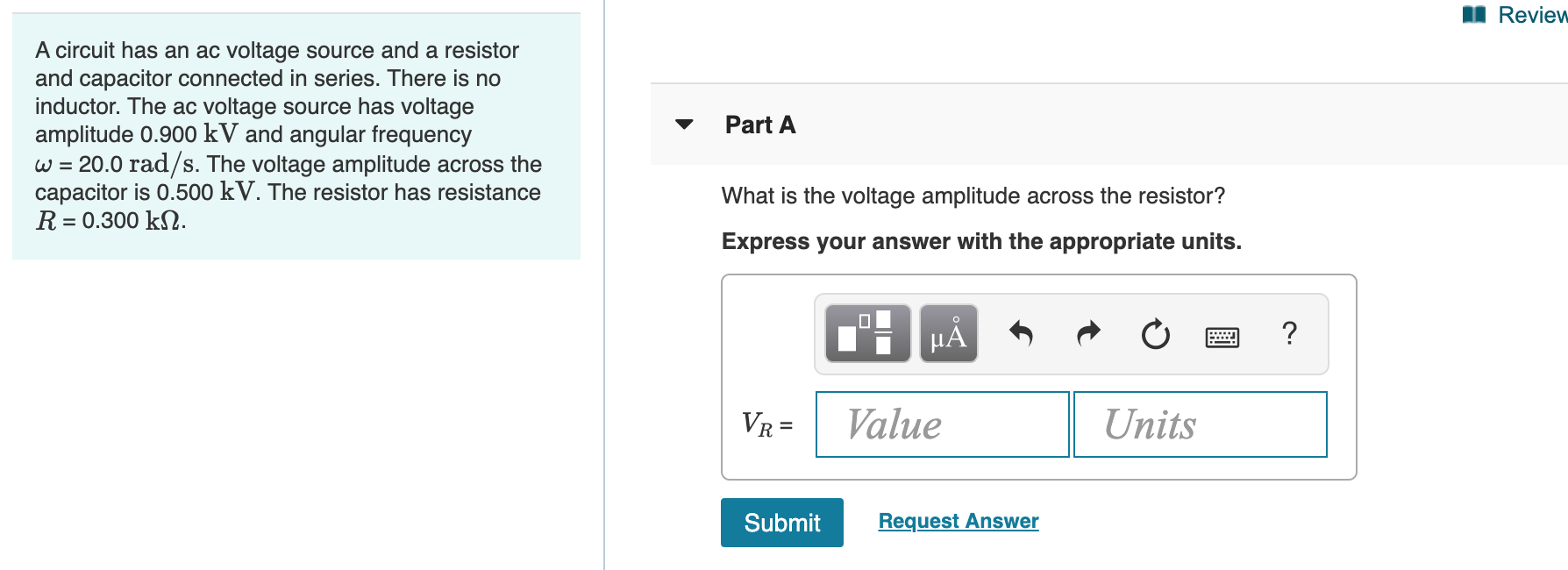Review A circuit has an ac voltage source and a resistor and capacitor connected in series. There is no inductor. The ac voltage source has voltage amplitude 0.900 kV and angular frequency w 20.0 rad/s. The voltage amplitude across the capacitor is 0.500 kV. The resistor has resistance R Part A What is the voltage amplitude across the resistor? = 0.300 k2. Express your answer with the appropriate units. HA ? Value Units VR= Request Answer Submit Part B What...

• ### I. A balanced three-phase positive sequence wye-connected 60Hz source voltage has line-neutral voltage of V, 1000 V. It is connected to a three- phase wye connected load whose impedance is z 50+j...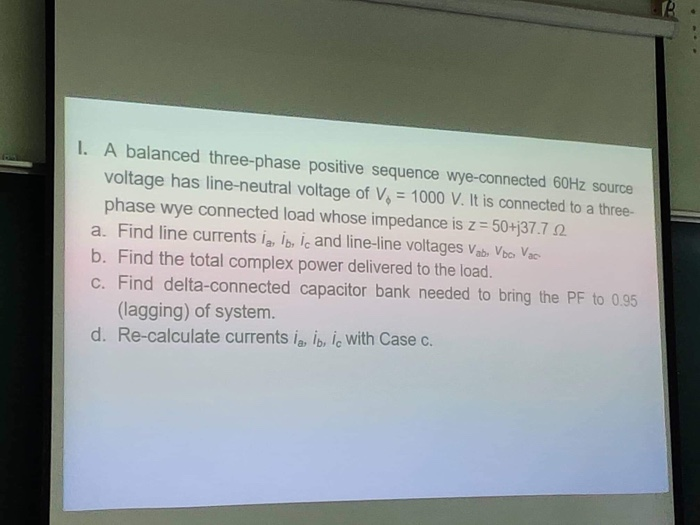I. A balanced three-phase positive sequence wye-connected 60Hz source voltage has line-neutral voltage of V, 1000 V. It is connected to a three- phase wye connected load whose impedance is z 50+j37.7 2 a. Find line currents ia ib, ic and line-line voltages Vab, Vbo Vac b. Find the total complex power delivered to the load. c. Find delta-connected capacitor bank needed to bring the PF to 0.95 (lagging) of system. d. Re-calculate currents la, ib, io with Case c....

• ### I. A balanced three-phase positive sequence wye-connected 60Hz source voltage has line-neutral voltage of V, 1000 V. It is connected to a three- phase wye connected load whose impedance is z 50+j...I. A balanced three-phase positive sequence wye-connected 60Hz source voltage has line-neutral voltage of V, 1000 V. It is connected to a three- phase wye connected load whose impedance is z 50+j37.7 2 a. Find line currents ia ib, ic and line-line voltages Vab, Vbo Vac b. Find the total complex power delivered to the load. c. Find delta-connected capacitor bank needed to bring the PF to 0.95 (lagging) of system. d. Re-calculate currents la, ib, io with Case c....

• ### Measures 480 V line-to-line Xline - 11.00 Total LOAD Load 1 Load 2 200 KVA @p.f....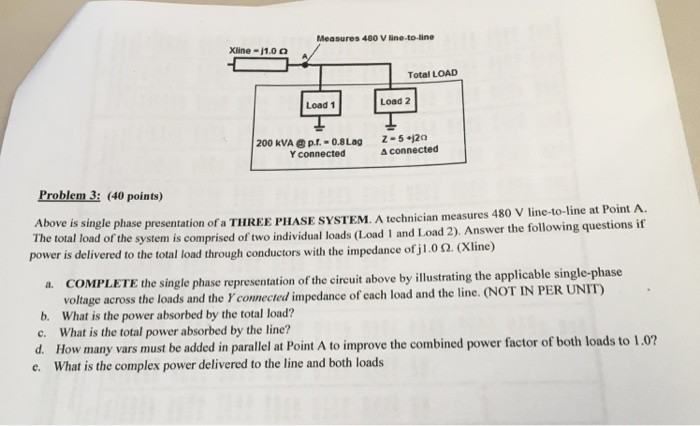Measures 480 V line-to-line Xline - 11.00 Total LOAD Load 1 Load 2 200 KVA @p.f. -0.8 Lag Y connected Z-5 20 A connected Problem 3: (40 points) Above is single phase presentation of a THREE PHASE SYSTEM. A technician measures 480 V line-to-line at Point A. The total load of the system is comprised of two individual loads (Load 1 and Load 2). Answer the following questions if power is delivered to the total load through conductors with the...

• ### 12. For a three-phase balanced system, a generator serves a remote load through two parallel tran...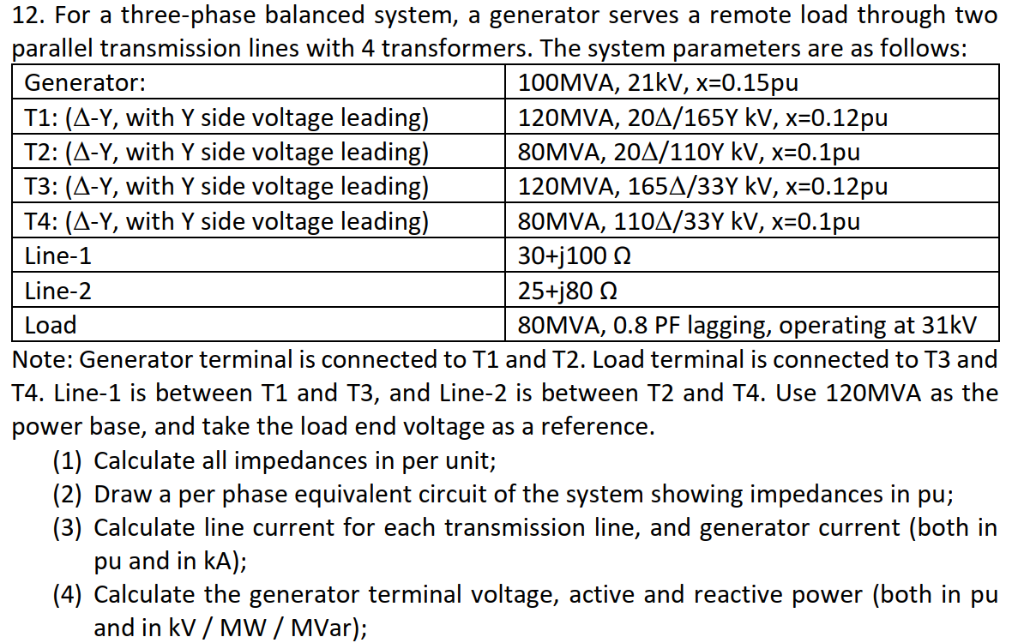12. For a three-phase balanced system, a generator serves a remote load through two parallel transmission lines with 4 transformers. The system parameters are as follows: Generator: T1: (A-Y, with Y side voltage leading) T2: (A-Y, with Y side voltage leading) T3: (A-Y, with Y side voltage leading) T4: (A-Y, with Y side voltage leading) Line-1 Line-2 Load 100MVA, 21kV, x-0.15pu 120MVA, 20Д/165Y kV, x-O.12pu 80MVA, 20A/1 10Y kV, x-O.1pu 120MVA, 165A/33Y kV, x-0.12pu 80MVA, 11ОД/33Y kV, x-O.1pu 30+j100 O...

• ### A 60-Hz single-phase AC power network is shown in the figure below with four different loads. The source voltage ES-320 0. Prior to adding the capacitor load for power factor correction, the source c...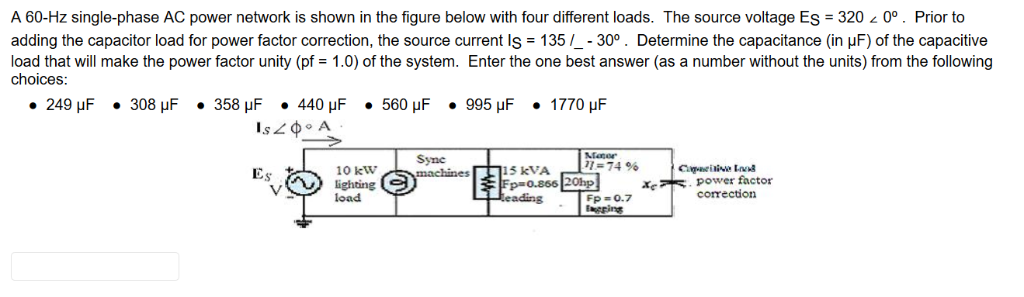A 60-Hz single-phase AC power network is shown in the figure below with four different loads. The source voltage ES-320 0. Prior to adding the capacitor load for power factor correction, the source current IS-135 30 . Determine the capacitance (in μF) of the capacitive load that will make the power factor unity (pf 1.0) of the system. Enter the one best answer (as a number without the units) from the following choices: Sync 74 % 6 15 kVA 10...

• ### 4) Calculate load voltage, phase, load current, phase and load power for the tables below. (fill ...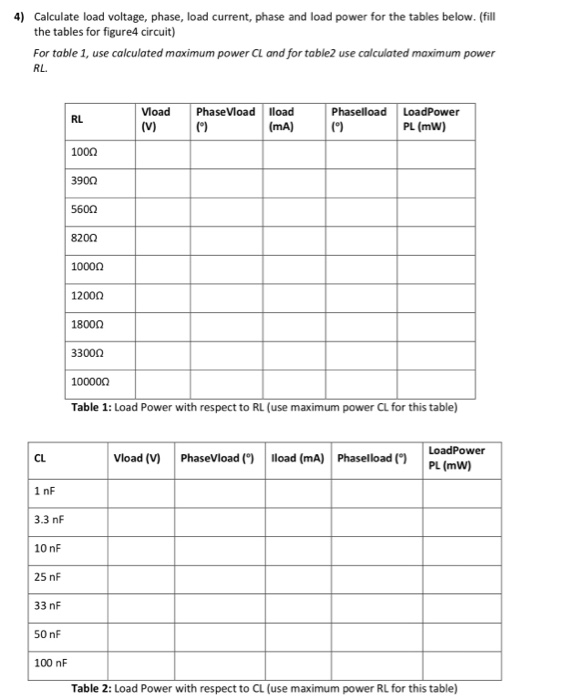4) Calculate load voltage, phase, load current, phase and load power for the tables below. (fill the tables for figure4 circuit) For table 1, use calculated maximum power CL and for table2 use calculated maximum power RL VoadPhaseVload lload (mA) RL PL (mW) 100Ω 3900 560Ω 8200 1000Ω 12000 1800Ω 3300Ω 1 0000Ω Table 1: Load Power with respect to RL (use maximum power CL for this table) LoadPower PL (mW) CL Vload (V)PhaseVload() Iload (mA) Phaselload() 1 nF 3.3...

Free Homework App